## TCP Congestion Control-

Before you go through this article, make sure that you have gone through the previous article on TCP Congestion Control.

TCP congestion control policy consists of following three phases-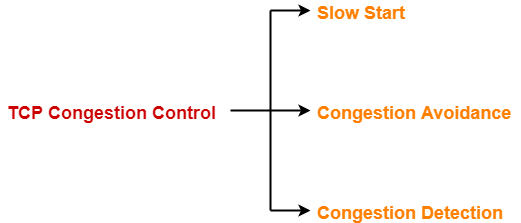1. Slow Start
2. Congestion Avoidance
3. Congestion Detection

In this article, we will discuss practice problems on TCP Congestion Control.

## Problem-01:

The growth of congestion window takes place-

1. Infinitely
2. Up to Threshold
3. Up to the size of receiver’s window
4. Up to timeout

## Solution-

Option (C) is correct.

## Problem-02:

Consider the effect of using slow start on a line with a 10 msec RTT and no congestion. The receiver window is 24 KB and the maximum segment size is 2 KB. How long does it take before the first full window can be sent?

## Solution-

Given-

• Receiver window size = 24 KB
• Maximum Segment Size = 2 KB
• RTT = 10 msec

Receiver window size in terms of MSS

= Receiver window size / Size of 1 MSS

= 24 KB / 2 KB

= 12 MSS

### Slow Start Threshold-

Slow start Threshold

= Receiver window size / 2

= 12 MSS / 2

= 6 MSS

### Slow Start Phase-

• Window size at the start of 1st transmission = 1 MSS
• Window size at the start of 2nd transmission = 2 MSS
• Window size at the start of 3rd transmission = 4 MSS
• Window size at the start of 4th transmission = 6 MSS

Since the threshold is reached, so it marks the end of slow start phase.

Now, congestion avoidance phase begins.

### Congestion Avoidance Phase-

• Window size at the start of 5th transmission = 7 MSS
• Window size at the start of 6th transmission = 8 MSS
• Window size at the start of 7th transmission = 9 MSS
• Window size at the start of 8th transmission = 10 MSS
• Window size at the start of 9th transmission = 11 MSS
• Window size at the start of 10th transmission = 12 MSS

From here,

• Window size at the end of 9th transmission or at the start of 10th transmission is 12 MSS.
• Thus, 9 RTT’s will be taken before the first full window can be sent.

So,

Time taken before the first full window is sent

= 9 RTT’s

= 9 x 10 msec

= 90 msec

## Problem-03:

Consider an instance of TCP’s Additive Increase Multiplicative Decrease (AIMD) algorithm where the window size at the start of slow start phase is 2 MSS and the threshold at the start of first transmission is 8 MSS. Assume that a time out occurs during the fifth transmission. Find the congestion window size at the end of tenth transmission.

1. 8 MSS
2. 14 MSS
3. 7 MSS
4. 12 MSS

## Solution-

Given-

• Window size at the start of slow start phase = 2 MSS
• Threshold at the start of first transmission = 8 MSS
• Time out occurs during 5th transmission

### Slow Start Phase-

• Window size at the start of 1st transmission = 2 MSS
• Window size at the start of 2nd transmission = 4 MSS
• Window size at the start of 3rd transmission = 8 MSS

Since the threshold is reached, so it marks the end of slow start phase.

Now, congestion avoidance phase begins.

### Congestion Avoidance Phase-

• Window size at the start of 4th transmission = 9 MSS
• Window size at the start of 5th transmission = 10 MSS

It is given that time out occurs during 5th transmission.

TCP reacts by-

• Setting the slow start threshold to half of the current congestion window size.
• Decreasing the congestion window size to 2 MSS (Given value is used).
• Resuming the slow start phase.

So now,

• Slow start threshold = 10 MSS / 2 = 5 MSS
• Congestion window size = 2 MSS

### Slow Start Phase-

• Window size at the start of 6th transmission = 2 MSS
• Window size at the start of 7th transmission = 4 MSS
• Window size at the start of 8th transmission = 5 MSS

Since the threshold is reached, so it marks the end of slow start phase.

Now, congestion avoidance phase begins.

### Congestion Avoidance Phase-

• Window size at the start of 9th transmission = 6 MSS
• Window size at the start of 10th transmission = 7 MSS
• Window size at the start of 11th transmission = 8 MSS

From here,

Window size at the end of 10th transmission

= Window size at the start of 11th transmission

= 8 MSS

Thus, Option (A) is correct.

## Problem-04:

Suppose that the TCP congestion window is set to 18 KB and a time out occurs. How big will the window be if the next four transmission bursts are all successful? Assume that the MSS is 1 KB.

## Solution-

### Congestion Window Size-

Congestion window size in terms of MSS

= 18 KB / Size of 1 MSS

= 18 KB / 1 KB

= 18 MSS

### Reaction Of TCP On Time Out-

TCP reacts by-

• Setting the slow start threshold to half of the current congestion window size.
• Decreasing the congestion window size to 1 MSS.
• Resuming the slow start phase.

So now,

• Slow start threshold = 18 MSS / 2 = 9 MSS
• Congestion window size = 1 MSS

### Slow Start Phase-

• Window size at the start of 1st transmission = 1 MSS
• Window size at the start of 2nd transmission = 2 MSS
• Window size at the start of 3rd transmission = 4 MSS
• Window size at the start of 4th transmission = 8 MSS
• Window size at the start of 5th transmission = 9 MSS

Thus, after 4 successful transmissions, window size will be 9 MSS or 9 KB.

## Problem-05:

On a TCP connection, current congestion window size is 4 KB. The window advertised by the receiver is 6 KB. The last byte sent by the sender is 10240 and the last byte acknowledged by the receiver is 8192.

### Part-01:

The current window size at the sender is ____.

1. 2048 B
2. 4096 B
3. 6144 B
4. 8192 B

### Part-02:

The amount of free space in the sender window is ____.

1. 2048 B
2. 4096 B
3. 6144 B
4. 8192 B

## Solution-

### Part-01:

Sender window size

= min (Congestion window size, Receiver window size)

= min(4KB , 6KB)

= 4 KB

= 4096 B

Thus, Option (B) is correct.

### Part-02:

Given-

• Last byte acknowledged by the receiver = 8192
• Last byte sent by the sender = 10240

From here,

• It means bytes from 8193 to 10240 are still present in the sender’s window.
• These bytes are waiting for their acknowledgement.
• Total bytes present in sender’s window = 10240 – 8193 + 1 = 2048 bytes.

From here,

Amount of free space in sender’s window currently

= 4096 bytes – 2048 bytes

= 2048 bytes

This indicates that half of the sender’s window is currently empty.

Thus, Option (A) is correct.

Next Article- TCP Timers

Get more notes and other study material of Computer Networks.

Watch video lectures by visiting our YouTube channel LearnVidFun.

## Problem-01:

How many TCP connections can be opened between two ports?

1. Multiple
2. Single
3. Zero
4. None

## Solution-

Option (B) is correct.

## Problem-02:

TCP protects itself from miss delivery by IP with the help of-

4. Source port and Destination port

## Solution-

Option (C) is correct.

## Problem-03:

What addressing system has topological significance?

## Solution-

Option (A) is correct.

## Problem-04:

If WAN link is 2 Mbps and RTT between source and destination is 300 msec, what would be the optimal TCP window size needed to fully utilize the line?

1. 60,000 bits
2. 75,000 bytes
3. 75,000 bits
4. 60,000 bytes

## Solution-

Given-

• Bandwidth = 2 Mbps
• RTT = 300 msec

### Optimal TCP Window Size-

Optimal TCP window size

= Maximum amount of data that can be sent in 1 RTT

= 2 Mbps x 300 msec

= 600 x 103 bits

= 60,0000 bits

= 75,000 bytes

Thus, Option (B) is correct.

## Problem-05:

Suppose host A is sending a large file to host B over a TCP connection. The two end hosts are 10 msec apart (20 msec RTT) connected by a 1 Gbps link. Assume that they are using a packet size of 1000 bytes to transmit the file. For simplicity, ignore ack packets. At least how big would the window size (in packets) have to be for the channel utilization to be greater than 80%?

1. 1000
2. 1500
3. 2000
4. 2500

## Solution-

Given-

• RTT = 20 msec
• Bandwidth = 1 Gbps
• Packet size = 1000 bytes
• Efficiency >= 80%

### Window Size For 100% Efficiency-

For 100% efficiency,

Window size

= Maximum number of bits that can be transmitted in 1 RTT

= 1 Gbps x 20 msec

= (109 bits per sec) x 20 x 10-3 sec

= 20 x 106 bits

= 2 x 107 bits

### Window Size For 80% Efficiency-

For 80% efficiency,

Window size

= 0.8 x 2 x 107 bits

= 1.6 x 107 bits

In terms of packets,

Window size

= 1.6 x 107 bits / Packet size

= 1.6 x 107 bits / (1000 x 8 bits)

= 0.2 x 104 packets

= 2000 packets

Thus, Option (C) is correct.

## Problem-06:

A TCP machine is sending windows of 65535 B over a 1 Gbps channel that has a 10 msec one way delay.

1. What is the maximum throughput achievable?
2. What is the line efficiency?

## Solution-

Given-

• Window size = 65535 bytes
• Bandwidth = 1 Gbps
• One way delay = 10 msec

### Method-01:

Maximum amount of data that can be sent in 1 RTT

= 1 Gbps x (2 x 10 msec)

= (109 bits per sec) x 20 x 10-3 sec

= 20 x 106 bits

= 25 x 105 bytes

Amount of data that is actually being sent in 1 RTT = 65535 bytes

Thus,

Line Efficiency(η)

= Amount of data being sent in 1 RTT / Maximum amount of data that can be sent in 1 RTT

= 65535 bytes / 25 x 105 bytes

= 0.026214

= 2.62%

Now,

Maximum Achievable Throughput

= Efficiency x Bandwidth

= 0.0262 x 1 Gbps

= 26.214 Mbps

### Method-02:

Maximum Achievable Throughput

= Number of bits sent per second

= 65535 B / 20 msec

= (65535 x 8 bits) / (20 x 10-3 sec)

= 26.214 Mbps

Now,

Line Efficiency

= Throughput / Bandwidth

= 26.214 Mbps / 1 Gbps

= 26.214 x 10-3

= 0.026214

= 2.62%

Next Article- TCP Congestion Control

Get more notes and other study material of Computer Networks.

Watch video lectures by visiting our YouTube channel LearnVidFun.

## Transport Layer Protocols-

There are mainly two transport layer protocols that are used on the Internet-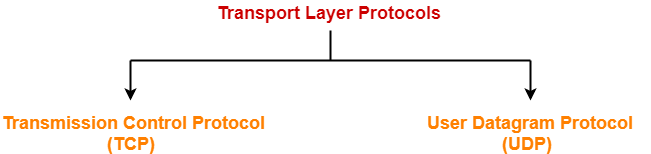1. Transmission Control Protocol (TCP)
2. User Datagram Protocol (UDP)

## UDP Protocol-

• UDP is short for User Datagram Protocol.
• It is the simplest transport layer protocol.
• It has been designed to send data packets over the Internet.
• It simply takes the datagram from the network layer, attaches its header and sends it to the user.

## Characteristics of UDP-

• It is a connectionless protocol.
• It is a stateless protocol.
• It is an unreliable protocol.
• It is a fast protocol.
• It offers the minimal transport service.
• It is almost a null protocol.
• It does not guarantee in order delivery.
• It does not provide congestion control mechanism.
• It is a good protocol for data flowing in one direction.

## Need of UDP-

• TCP proves to be an overhead for certain kinds of applications.
• The Connection Establishment Phase, Connection Termination Phase etc of TCP are time consuming.
• To avoid this overhead, certain applications which require fast speed and less overhead use UDP.

The following diagram represents the UDP Header Format-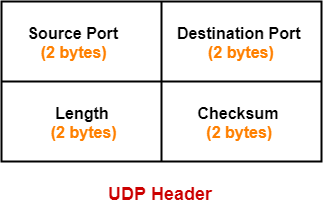## 1. Source Port-

• Source Port is a 16 bit field.
• It identifies the port of the sending application.

## 2. Destination Port-

• Destination Port is a 16 bit field.
• It identifies the port of the receiving application.

## 3. Length-

• Length is a 16 bit field.
• It identifies the combined length of UDP Header and Encapsulated data.

 Length = Length of UDP Header + Length of encapsulated data

## 4. Checksum-

• Checksum is a 16 bit field used for error control.
• It is calculated on UDP Header, encapsulated data and IP pseudo header.
• Checksum calculation is not mandatory in UDP.

## Applications Using UDP-

Following applications use UDP-

• Applications which require one response for one request use UDP. Example- DNS.
• Routing Protocols like RIP and OSPF use UDP because they have very small amount of data to be transmitted.
• Trivial File Transfer Protocol (TFTP) uses UDP to send very small sized files.
• Broadcasting and multicasting applications use UDP.
• Streaming applications like multimedia, video conferencing etc use UDP since they require speed over reliability.
• Real time applications like chatting and online games use UDP.
• Management protocols like SNMP (Simple Network Management Protocol) use UDP.
• Bootp / DHCP uses UDP.
• Other protocols that use UDP are- Kerberos, Network Time Protocol (NTP), Network News Protocol (NNP), Quote of the day protocol etc.

## Note-01:

 Size of UDP Header= 8 bytes

• This is because in UDP header, all the fields are of definite size.
• Size of UDP Header = Sum of the size of all the fields = 8 bytes.

## Note-02:

 UDP is almost a null protocol.

This is because-

• UDP provides very limited services.
• The only services it provides are checksumming of data and multiplexing by port number.

## Note-03:

 UDP is an unreliable protocol.

This is because-

• UDP does not guarantee the delivery of datagram to its respective user (application).
• The lost datagrams are not retransmitted by UDP.

## Note-04:

 Checksum calculation is not mandatory in UDP.

This is because-

• UDP is already an unreliable protocol and error checking does not make much sense.
• Also, time is saved and transmission becomes faster by avoiding to calculate it.

It may be noted-

• To disable the checksum, the field value is set to all 0’s.
• If the computed checksum is zero, the field value is set to all 1’s.

## Note-05:

 UDP does not guarantee in order delivery.

This is because-

• UDP allows out of order delivery to ensure better performance.
• If some data is lost on the way, it does not call for retransmission and keeps transmitting data.

## Note-06:

 Application layer can perform some tasks through UDP.

Application layer can do the following tasks through UDP-

1. Trace Route
2. Record Route
3. Time stamp

When required,

• Application layer conveys to the UDP which conveys to the IP datagram.
• UDP acts like a messenger between the application layer and the IP datagram.

## Problem-01:

Which field is optional in UDP?

1. Checksum
2. Destination port
3. Length
4. None

## Solution-

• Checksum calculation is not mandatory in UDP.
• Thus, Option (A) is correct.

## Problem-02:

The pseudo header of IP is used in-

1. Only TCP
2. Only UDP
3. Both TCP and UDP
4. None

## Solution-

• IP Pseudo header is used in both TCP and UDP while calculating checksum.
• Thus, Option (C) is correct.

## Problem-03:

Broadcasting applications like WHOD (who daemon on UNIX) uses what transport layer protocol?

1. TCP
2. UDP
3. Either TCP or UDP
4. IGMP

## Solution-

• Broadcasting and multicasting applications use UDP.
• Thus, Option (B) is correct.

Watch this Video Lecture

Next Article- Application Layer Protocols

Get more notes and other study material of Computer Networks.

Watch video lectures by visiting our YouTube channel LearnVidFun.

## Three Way Handshake-

Before you go through this article, make sure that you have gone through the previous article on Three Way Handshake.

We have discussed-

• TCP uses Three Way Handshake to establish a connection between the sender and receiver.
• Connection establishment using Three Way Handshake involves the steps as shown-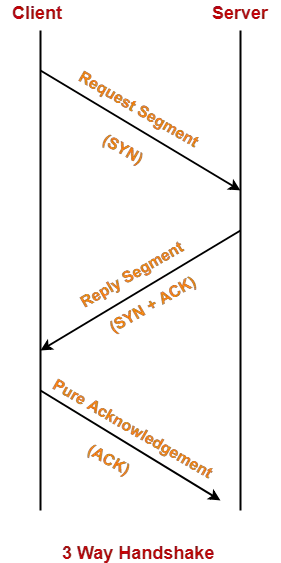In this article, we will discuss how a lost TCP segment is retransmitted.

## TCP Retransmission-

After establishing the connection,

• Sender starts transmitting TCP segments to the receiver.
• A TCP segment sent by the sender may get lost on the way before reaching the receiver.
• This causes the receiver to send the acknowledgement with same ACK number to the sender.
• As a result, sender retransmits the same segment to the receiver.
• This is called as TCP retransmission.

## When TCP Retransmission Occurs?

 When sender discovers that the segment sent by it is lost,it retransmits the same segment to the receiver.

Sender discovers that the TCP segment is lost when-

1. Either Time Out Timer expires
2. Or it receives three duplicate acknowledgements

## 1. Retransmission After Time Out Timer Expiry-

Each time sender transmits a TCP segment to the receiver, it starts a Time Out Timer.

Now, following two cases are possible-

### Case-01:

• Sender receives an acknowledgement for the sent segment before the timer goes off.
• In this case, sender stops the timer.

### Case-02:

• Sender does not receives any acknowledgement for the sent segment and the timer goes off.
• In this case, sender assumes that the sent segment is lost.
• Sender retransmits the same segment to the receiver and resets the timer.

## Example-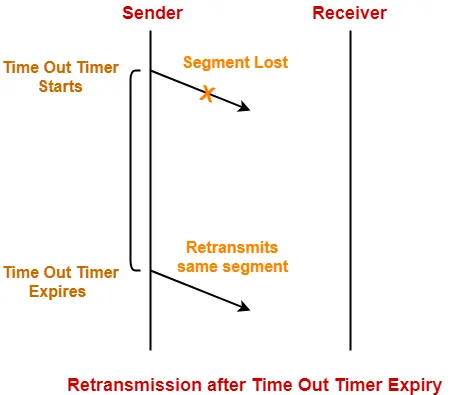## 2. Retransmission After Receiving 3 Duplicate Acknowledgements-

• Consider sender receives three duplicate acknowledgements for a TCP segment sent by it.
• Then, sender assumes that the corresponding segment is lost.
• So, sender retransmits the same segment without waiting for its time out timer to expire.
• This is known as Early retransmission or Fast retransmission.

## Example-

Consider-

• Sender sends 5 TCP segments to the receiver.
• The second TCP segment gets lost before reaching the receiver.

The sequence of steps taking place are-

(Original ACK)

(1st duplicate ACK)

(2nd duplicate ACK)

(3rd duplicate ACK)

Now,

• Sender receives 3 duplicate acknowledgements for segment-2 in total.
• So, sender assumes that the segment-2 is lost.
• So, it retransmits segment-2 without waiting for its timer to go off.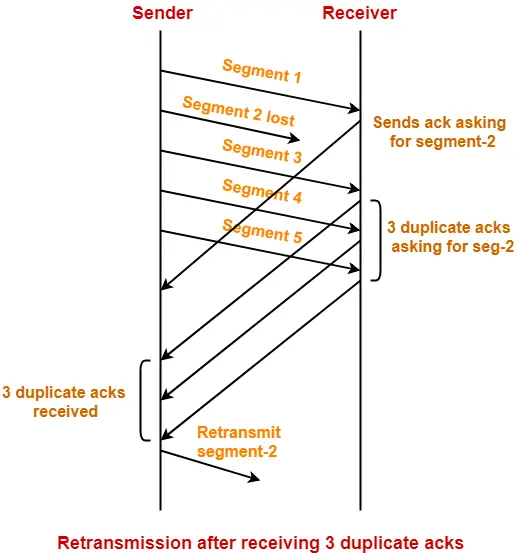## NOTE

After receiving the retransmitted segment-2,

• Receiver does not send the acknowledgement asking for segment-3 or 4 or 5.
• Receiver sends the acknowledgement asking for segment-6 directly from the sender.
• This is because previous segments have been already received and acknowledgements for them have been already sent (although wasted in asking for segment-2).

## Important Points-

### Point-01:

• Consider time out timer expires before receiving the acknowledgement for a TCP segment.
• This case suggests the stronger possibility of congestion in the network.

### Point-02:

• Consider sender receives 3 duplicate acknowledgements for the same TCP segment.
• This case suggests the weaker possibility of congestion in the network.

### Point-03:

• Consider receiver does not receives 3 duplicate acknowledgements for the lost TCP segment.
• In such a case, retransmission occurs only after time out timer goes off.

### Point-04:

• Retransmission on receiving 3 duplicate acknowledgements is a way to improve the performance over retransmission on time out.

## Problem-

Consider a TCP connection in a state where there are no outstanding ACKs. The sender sends two segments back to back. The sequence numbers of the first and second segments are 230 and 290 respectively. The first segment was lost but the second segment was received correctly by the receiver.

Let X be the amount of data carried in the first segment (in bytes) and Y be the ACK number sent by the receiver.

The values of X and Y are-

1. 60 and 290
2. 230 and 291
3. 60 and 231
4. 60 and 230

## Solution-

It is given that sender sends two segments where-

• 1st segment contains the sequence number 230 and gets lost.
• 2nd segment contains the sequence number 290 and is received correctly.

### Amount Of Data Sent In First Segment-

Given-

• Sequence number of 1st segment = 230
• Sequence number of 2nd segment = 290

From here, range of sequence numbers contained in the 1st segment = [230,289].

Now,

• Total number of sequence numbers contained in the 1st segment = 289 – 230 + 1 = 60.
• TCP assigns one sequence number to each byte of data.
• Thus, Amount of data contained in the first segment = 60 bytes.

### ACK Number Sent By Receiver-

On receiving the 2nd segment,

• This is because it expects the 1st segment first.
• Receiver keeps sending this ACK number until it receives the first segment correctly.
• Thus, Acknowledgement number = Sequence number of the 1st segment = 230.

Thus, Option (D) is correct.

Next Article- TCP Connection Termination

Get more notes and other study material of Computer Networks.

Watch video lectures by visiting our YouTube channel LearnVidFun.

## Transmission Control Protocol-

Before you go through this article, make sure that you have gone through the previous article on TCP in Networking.

We have discussed-

• TCP continuously receives data from the application layer.
• It divides the data into chunks where each chunk is a collection of bytes.
• It then creates TCP segments by adding a TCP header to the data chunks.

 TCP segment = TCP header + Data chunk

## TCP Sequence Number-

• Each TCP segment sent by the sender contains some bytes of data.
• TCP assigns a unique number to each data byte for its identification.
• This unique number is called as TCP Sequence Number.

## Purpose-

Sequence number serves the following purposes-

• It helps to identify each data byte uniquely.
• It helps in the segmentation of data into TCP segments and reassemble them later.
• It helps to keep track of how much data has been transferred and received.
• It helps to put the data back into the correct order if it is received in the wrong order.
• It helps to request data when it has been lost in transit.

## Maximum Number of Sequence Numbers-

• In TCP header, sequence number is a 32 bit field.
• So, maximum number of possible sequence numbers = 232.
• These sequence numbers lie in the range [0 , 232 – 1].

## NOTE-

• Maximum number of possible sequence numbers = 232.
• This does not imply that only 232 bytes = 4 GB data can be sent using TCP.
• The concept of wrap around allows to send unlimited data using TCP.

## Concept Of Wrap Around-

The concept of wrap around states-

 After all the 232 sequence numbers are used up and more data is to be sent,the sequence numbers can be wrapped around and used again from the starting.

In general,

• If the initial sequence number chosen is X.
• Then sequence numbers are used from X to 232 – 1 and then from to 0 to X-1.
• Then, sequence numbers are wrapped around to send more data.

## Example-

• Consider the initial sequence number used is 0.
• Then after sending 4 GB data, all the sequence numbers would get used up.
• To send more data, sequence numbers are reused from the starting.
• Wrapping around can be done again and again to send more and more data.

## Wrap Around Time-

• Time taken to use up all the 232 sequence numbers is called as wrap around time.
• It depends on the bandwidth of the network i.e. the rate at which the bytes go out.
• More the bandwidth, lesser the wrap around time and vice versa.

 Wrap Around Time ∝ 1 / Bandwidth

## Formula-

If bandwidth of the network = x bytes/sec, then-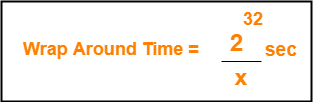## Life Time Of TCP Segment-

In modern computers,

• Life time of a TCP segment is 180 seconds or 3 minutes.
• It means after sending a TCP segment, it might reach the receiver taking 3 minutes in the worst case.

## How Wrap Around Is Possible?

It is possible to wrap around the sequence numbers because-

• The life time of a TCP segment is just 180 seconds.
• Wrap around time is much greater than life time of a TCP segment.
• So, by the time the sequence numbers wrap around, there is no probability of existing any segment having the same sequence number.
• Thus, even after wrapping around, the sequence number of all the bytes will be unique at any given time.

## Reducing Wrap Around Time-

 Wrap around time can be reduced to the life time of a TCP segment.

This is because-

• After the life time of a segment completes, it is considered that the segment no longer exists.
• So, sequence numbers used by the segment frees up and can be reused.

To reduce the wrap around time to the life time of segment,

• There must exist as many sequence numbers as there are number of data bytes sent in time equal to life time of segment.

## Formula-

 Number of bits required in the sequence number fieldso that wrap around time becomes equal to lifetime of TCP segment= log2 (lifetime of TCP segment x Bandwidth)

• The number of bits will be greater than 32 bits.
• The extra bits are appended in the Options field of TCP header.

## Problem-01:

Given the bandwidth of a network is 1 MB / sec. Calculate the wrap around time.

## Solution-

We know,

• Wrap around time = Time taken to use all the 232 sequence numbers.
• TCP assigns 1 sequence number to each byte of data.

To calculate wrap around time, we just need to calculate how much time will be taken to send 232 bytes of data.

Now,

• Given bandwidth = 1 MB / sec = 106 bytes / sec.
• It means 106 bytes of data is sent in time = 1 sec.
• So, 232 bytes of data will be sent in time = ( 1 / 106 ) x 232 sec.
• On solving, we get 1.19 hours.

Thus,

• It will take 1.19 hours to consume all the 232 sequence numbers if bandwidth = 1 MB / sec.
• Wrap Around Time = 1.19 hours.

Alternatively,

Using the formula, we have-

Wrap Around Time

= 232 / 106 sec

= 1.19 hours

## Problem-02:

If bandwidth of the network is 1 GBps, how many extra bits will have to be appended in the Options field so that wrap around time becomes equal to the life time of segment?

## Solution-

For wrap around time to become equal to the life time of TCP segment,

Number of sequence numbers required = Number of bytes sent in life time of TCP segment

We know-

• Life time of TCP segment = 180 sec.
• Bandwidth of the network = 1 GBps (Given)

Now,

• Number of bytes transferred in 1 sec = 1 GB
• So, number of bytes transferred in 180 sec = 180 GB = 180 x 230 bytes
• So, number of sequence numbers required = 180 x 230

Suppose y number of bits in the sequence number field are required to represent the value 180 x 230.

So, we have-

2y = 180 x 230

ylog2 = log(180 x 230)

y = log2(180 x 230)

y = log2180 + log2230

y = 7.49 + 30

y ≅ 38

From here,

• Total number of bits required for sequence numbers = 38 bits.
• In TCP header, sequence number field is a 32 bit field.
• So, extra bits required to be appended in the Options field = 38 – 32 = 6 bits.

Alternatively,

Using the formula, we have-

Total bits required

= log2 (life time of TCP segment x bandwidth)

= log2 ( 180 x 230)

= log2180 + log2230

= 7.49 + 30

= 38

From here,

• Total number of bits required for sequence numbers = 38 bits.
• In TCP header, sequence number field is a 32 bit field.
• So, extra bits required to be appended in the Options field = 38 – 32 = 6 bits.

## Problem-03:

In a network that has a maximum TPDU size of 128 bytes, a maximum TPDU lifetime of 30 sec and 8 bit sequence number, what is the maximum data rate per connection?

(TPDU is Transport layer protocol data unit which is the segment.)

## Solution-

Given-

• Maximum segment size (MSS) = 128 bytes
• Segment lifetime = 30 sec
• Bits in sequence number = 8

Now,

• Maximum number of possible sequence numbers using 8 bits = 28 = 256.
• So, maximum number of bytes that can be uniquely identified = 256 bytes.
• Lifetime of a segment = 30 seconds.
• So, maximum amount of data that can be sent in 30 seconds = 256 bytes.

Thus,

Maximum data rate per connection

= 256 bytes / 30 seconds

≈ 68 bits/sec

## Problem-04:

Suppose that the advertised window 1 MB long. If a sequence number is selected at random from the entire sequence number space, what is the probability that the sequence number falls inside the advertised window?

## Solution-

We know,

• Number of bits in sequence number field = 32 bits.
• So, Maximum number of sequence numbers possible = 232.
• 232 bytes of data can be labeled uniquely with these sequence numbers.
• Advertised window size = 1 MB = 220 bytes which uses 220 sequence numbers.

Therefore,

Required probability

= 220 / 232

= 1 / 212

Next Article- Three Way Handshake

Get more notes and other study material of Computer Networks.

Watch video lectures by visiting our YouTube channel LearnVidFun.